# BEE Basic Electricity Electronics question paper December 2014

BEE Basic Electricity Electronics question paper December 2014 – The semester 1 B.E.E question paper for Mumbai university F.E first year students is shown as follows:

### BEE Basic Electricity Electronics question paper December 2014

1(a) Convert the given circuit into a single current source in parallel with a single resistance between points A and B.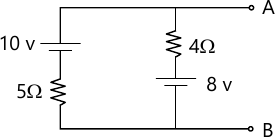3 M
1(b) Find voltage VAB using super position theorem.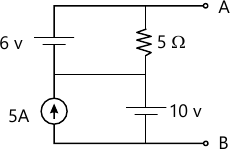3 M
1(c) Find the average value of the following waveform.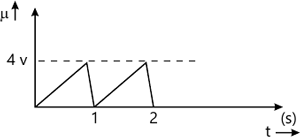3 M
1(d) For a series RLC circuit having R=10&Omega, L=0.01 H and C=100?F, find the resonant frequency, quality factor and bandwidth.
3 M
1(e) Draw the phasor diagram for 3-phase star connected load with a leading power factor. Indicate line and phase voltage and currents.
2 M
1(f) A 5kVA, 240/2400 V, 50Hz single phase transformer has the maximum value of flu density at 1.2 Tesla. If the e.m.f. per turn is 8V. Calculate the number of primary and secondary turns and the primary and secondary current at full load.
4 M
1(g) Draw the cicruit diagram and the input and output waveforms for a full wave bridge rectifier.
2 M
2(a) Find the current through 4? resistance using Nodal analysis.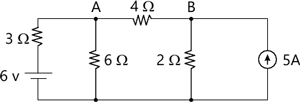6 M
2(b) Two coils are connected in series across a 200V, 50 Hz AC supply. The power input to the circuit is 2kW and 1.1kVAR. If the resistance and reactance of the first coil are 5? and 8? respectively calculate the resistance and reactance of the second coil. Calculate the active power and reactive power for both the coils individually.
8 M
2(c) Explain the principle of working for a single phase transformer and derive the e.m.f. equation for the same.
6 M
3(a) A balanced 3-phase load consists of 3 coils, each of resistance 4? and inductance 0.02H. It is connected to a 440V, 50 Hz 3? supply. Find the total power consumed when the load is connected in star and the total reactive power when the load is connected in delta.
8 M
3(b) With the help of a neat diagram explain how open circuit test is conducted on a single phase transformer.
6 M
3(c) Draw the circuit diagram of a half wave rectifier with the capacitor filter and the corresponding input and output waveforms for the same.
2 M
3(d) With the help of a neat diagram explain the input waveform characteristics of an NPN transistor in the common emitter configuration.
4 M
4(a) Using source transformation find the current flowing through the 10? resistance.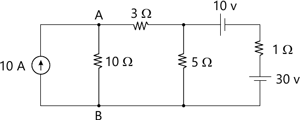7 M
4(b) Find the R.M.S. value for the given waveform.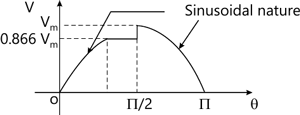5 M
4(c) Two wattmeters are used to measure power in a 3-phase balanced star connected load using the two wattmeter method. The readings of the 2 wattmeters are 8kW and 4kW respectively. Calculate the total power consumed by the 3-phase load and the power factor.
4 M
4(d) With the hlp of neat circuit diagram and input and output waveforms explain the working of a fullwave centre tapped rectifier.
4 M
5(a) For the given circuit find the value of RL for maximum power transfer and calculate the maximum power absorbed by RL.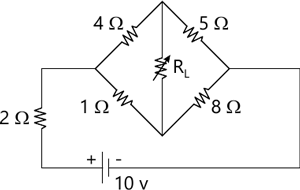8 M
5(b) The voltage and current in a circuit are given by e=100sin (?t+30°) and i=50sin(?t+60°). Determine the impedance of th ecircuit. Assuming the circuit to contain 2 elements in series find resistance, reactance and power factor of the circuit.
4 M
5(c) A 30kVA, 2400/120V, 50Hz transformer has high-voltage winding resistance of 0.1? and leakage reactance of 0.22?. The low voltage winding resistance is 0.035&Omega and leakage reactance s 0.012?. Calculate equivalent resistance as referred to primary and secondary, equivalent reactance as referred to primary and secondary, equivalent impedance as referred to primary and secondary. Copper loss at full load and at 75% of full load.
8 M
6(a)Find the value of current flowing through the 5? resistance using Superposition Theorem.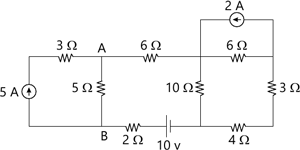7 M
6(b) An inductive coil having a resistance of 20? and inductance of 0.2H is connected in parallel with a 20?F capacitor with variable frequency and 230V supply. Find the frequency at which the total current draw from supply is in phase with the supply voltage. Find the value of the current and the impedance of the circuit at this frequency.
7 M
6(c) With the help of a neat circuit diagram and phasor diagram. Explain the 2-wattmeter method to measure power in a 3-phase balanced delta connected load.
6 M